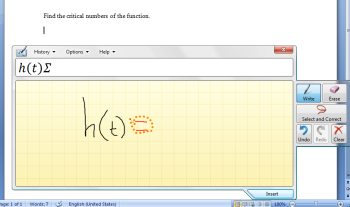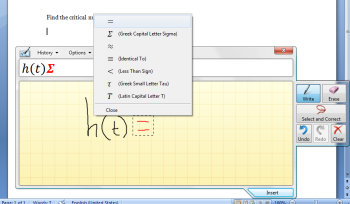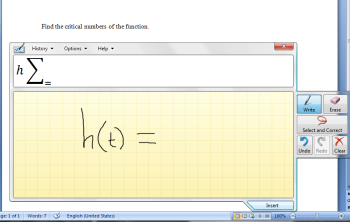# Microsoft Math Input Panel Cannot Produce Equal Sign

UPDATE: The problem was that I was not completing the equation. When I wrote out the full equation, Math Input Panel did, in fact, correctly recognize the equal sign.

I wanted to end up with this equation:$h(t)=t^{\frac{3}{4}}\text{--}2t^{\frac{1}{4}}$

When I wrote the equal sign, I got a capital sigma:So, I tried to use the correction feature.The equal sign pops up as an option, but, when selected, the resulting equation is nonsense:This site uses Akismet to reduce spam. Learn how your comment data is processed.